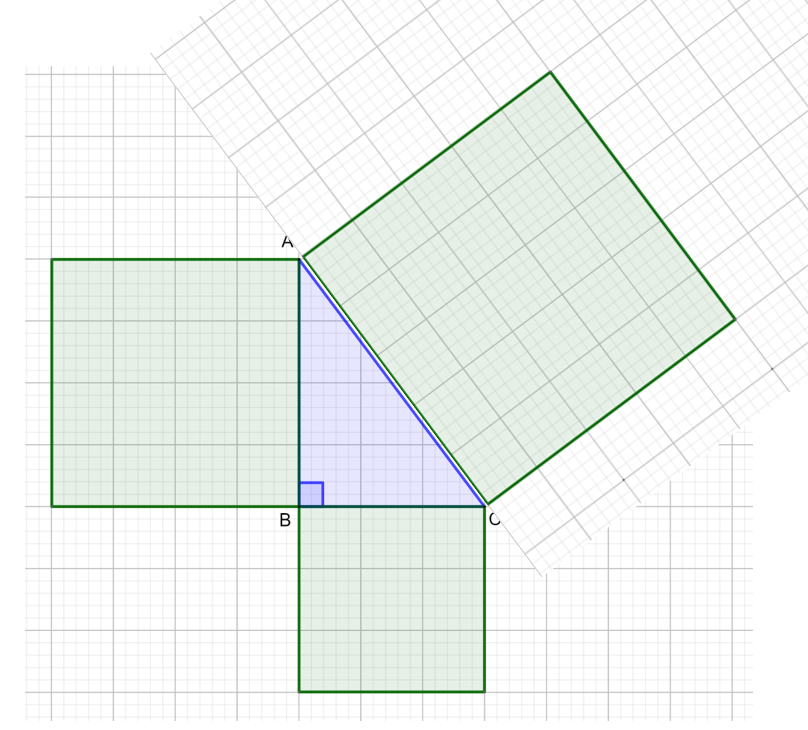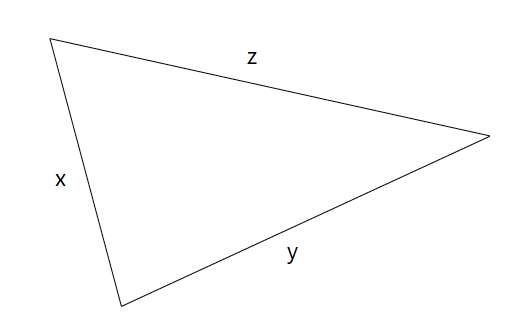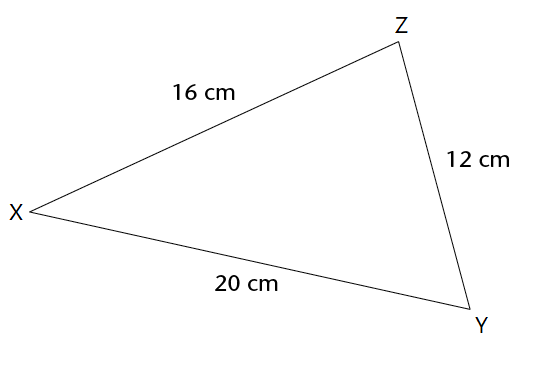Home Practice
For learners and parents For teachers and schools
Textbooks
Full catalogue
Pricing SupportLog in

We think you are located in United States. Is this correct?

# Theorem of Pythagoras

## 15.4 Theorem of Pythagoras

The theorem of Pythagoras is a rule that applies only to right-angled triangles. The side opposite the right angle of a right-angled triangle is called the hypotenuse. The hypotenuse is always the longest side of a right-angled triangle.

theorem
a rule or statement that has been proved through logical reasoning
hypotenuse
the side opposite the right angle in a right-angled triangle

### Investigate the theorem of Pythagoras

For this investigation, you will need a pencil, a ruler, a pair of scissors and two pieces of squared paper.

Read through all the instructions before you start the investigation.

### Part 1: Draw $$\triangle ABC$$ and three squares

1. Fold one piece of squared paper in half so that you have a top section and a bottom section.
2. In the top section, draw $$\triangle ABC$$ with $$\angle B = 90^{\circ}$$, $$AB = 4 \text{ units}$$ and $$BC = 3 \text{ units}$$.
3. Which side of the triangle is the hypotenuse?
4. On your diagram, draw a square using $$AB = 4 \text{ units}$$ as one of the sides (see diagram below).
5. What is the area of square 1?
6. Draw a second square using $$BC = 3 \text{ units}$$ as one of the sides.
7. What is the area of square 2?8. Cut the other piece of squared paper in half.
9. Turn the piece of paper and align the squares with side $$AC$$ (you can use some glue to keep the piece of paper in position).
10. How many units long is side $$AC$$?
11. Draw a third square using $$AC$$ as one of the sides.
12. What is the area of square 3?13. Use your diagram to complete the table for the area of each square:

Area Square 1 Area Square 2 Area Square 1 + Area Square 2 Area Square 3

14. Use your diagram to complete the table for the lengths of the sides of the triangle:

$$AB$$ $$BC$$ $$AC$$ $$AB^2 + BC^2$$ $$AC^2$$

15. What do you notice about the last two columns in the tables?

### Part 2: Draw $$\triangle DEF$$ and three squares

1. In the bottom section of the squared paper, draw $$\triangle DEF$$ with $$\angle E = 90^{\circ}$$, $$DE = 5 \text{ units}$$ and $$EF = 12 \text{ units}$$.
2. Which side of the triangle is the hypotenuse?
3. On your diagram, draw a square using $$DE = 5 \text{ units}$$ as one of the sides.
4. What is the area of square 1?
5. Draw a second square using $$EF = 12 \text{ units}$$ as one of the sides.
6. What is the area of square 2?
7. Turn another piece of squared paper and align the squares with side $$DF$$.
8. How many units is side $$DF$$?
9. Draw a third square using $$DF$$ as one of the sides.
10. What is the area of square 3?
11. Use your diagram to complete the table for the area of each square:

Area Square 1 Area Square 2 Area Square 1 + Area Square 2 Area Square 3

12. Use your diagram to complete the table for the lengths of the sides of the triangle:

$$DE$$ $$EF$$ $$DF$$ $$DE^2 + EF^2$$ $$DF^2$$

13. What do you notice about the last two columns in the tables?
14. What can you conclude from this investigation?
15. Complete the sentence: In a right-angled triangle, the square of the hypotenuse is equal to …
16. $$\triangle XYZ$$ is a right-angled triangle with hypotenuse $$x$$ and sides $$y$$ and $$z$$. Which of the following is the correct formula for the theorem of Pythagoras?
• $$y^2 = x^2 + z^2$$
• $$x^2 = z^2 + y^2$$
• $$z^2 = y^2 + x^2$$

### The theorem of Pythagoras

In a right-angled triangle, the square of the hypotenuse is equal to the sum of the squares of the two other sides.

$$c^2 = a^2 + b^2$$ or $$a^2 + b^2 = c^2$$• In a right-angled triangle, we can use the theorem of Pythagoras to calculate the unknown length of a side.
• If we can show that $$c^2 = a^2 + b^2$$ for the lengths of the sides of a triangle, then the triangle is a right-angled triangle.

### Using the theorem of Pythagoras to prove a triangle is a right-angled triangle

If we know the lengths of all three sides of a triangle, we can use the theorem of Pythagoras to determine if the triangle is a right-angled triangle or not.

For the triangle below, $$z$$ is the longest side of the triangle.• If $$z^2 = x^2 + y^2$$, then the theorem of Pythagoras holds true and the triangle is a right-angled triangle.
• If $$z^2$$ is not equal to $$x^2 + y^2$$, then the theorem of Pythagoras does not hold true and the triangle is not a right-angled triangle.

## Worked Example 15.7: Using the theorem of Pythagoras to prove a triangle is a right-angled triangle

Use the theorem of Pythagoras to show that $$\triangle XYZ$$ is a right-angled triangle.### Identify the longest side of the triangle.

In $$\triangle XYZ$$, $$XY = 20 \text{ cm}, YZ= 12 \text{ cm}$$ and $$ZX = 16 \text{ cm}$$. So the longest side is $$XY$$, which means that if $$\triangle XYZ$$ is a right-angled triangle, then $$XY$$ is the hypotenuse.

### Calculate $$XY^2$$ and $$YZ^2 + ZX^2$$.

In $$\triangle XYZ$$,

\begin{align} XY^2 &= 20^2 \\ &= 400 \end{align} \begin{align} YZ^2 + ZX^2 &= 12^2 + 16^2 \\ &= 144 + 256 \\ &= 400 \end{align}

We have shown that $$XY^2 = YZ^2 + ZX^2$$

This means that the theorem of Pythagoras holds true, so $$\triangle XYZ$$ is a right-angled triangle.

$$\triangle XYZ$$ is a right-angled triangle with $$\angle Z = 90^{\circ}$$.

Exercise 15.3

Use the theorem of Pythagoras to determine if the triangle is a right-angled triangle or not.$$c^2 = a^2 + b^2$$ (Pythagoras)

LHS $$= c^2 = 6^2 = 36$$

RHS $$= a^2 + b^2 = 4^2 + 4^2 = 16 + 16 = 32$$

Not a right-angled triangle

A triangle with sides $$4 \text{ units}, 15 \text{ units}$$ and $$12 \text{ units}$$ is shown. Is this a right-angled triangle? Show your calculations.$$c^2 = a^2 + b^2$$ (Pythagoras)

LHS: $$c^2 = 15^2 = 225$$

RHS: $$a^2 + b^2 = 4^2 + 12^2 = 16 + 144 = 160$$

Not a right-angled triangle

In each case the lengths of the three sides of a triangle are given. Determine whether the triangle is a right-angled triangle or not.

1. $$7, 9$$ and $$12$$
2. $$16, 8$$ and $$10$$
3. $$7, 12$$ and $$14$$
4. $$10, 8$$ and $$6$$
5. $$15, 17$$ and $$8$$
6. $$16, 25$$ and $$21$$
1. Not right-angled triangle
2. Not right-angled triangle
3. Not right-angled triangle
4. Right-angled triangle
5. Right-angled triangle
6. Not right-angled triangle

Use the theorem of Pythagoras to determine if the triangle is a right-angled triangle or not.$$c^2 = a^2 + b^2$$ (Pythagoras)

LHS $$= c^2 = 7^2 = 49$$

RHS $$= a^2 + b^2 = 3^2 + 5^2 = 9 + 25 = 34$$

Not a right-angled triangle

## Worked Example 15.8: Calculating the length of the hypotenuse in a right-angled triangle

In $$\triangle KLM$$, $$\angle L = 90^{\circ}$$, $$KL = 8 \text{ cm}$$ and $$LM = 6 \text{ cm}$$.

Determine the length of $$KM$$. Leave your answer in its simplest form.### Use the theorem of Pythagoras to find $$KM$$.

$$\triangle KLM$$ is a right-angled triangle, so we can use the theorem of Pythagoras to find the length of $$KM$$. $$KM$$ lies opposite the right angle, so $$KM$$ is the hypotenuse.

\begin{align} KM^2 &= KL^2 + LM^2 \ (\text{Pythagoras}) \\ &= 8^2 + 6^2 \\ &= 64 + 36 \\ &= 100 \\ \therefore KM &= \sqrt{100} \\ &= 10 \end{align}

$KM = 10 \text{ cm}$

## Worked Example 15.9: Calculating the length of an unknown side in a right-angled triangle

Find $$y$$. Give your answer in simplest surd form.### Use the theorem of Pythagoras to find $$y$$.

In this right-angled triangle, the hypotenuse is the side of length $$20 \text{ units}$$.

$20^2 = y^2 + 15^2$

Rearrange the equation to determine $$y$$:

\begin{align} 20^2 − 15^2 &= y^2 \\ 400\ –\ 225 &= y^2 \\ 175 &= y^2 \end{align}

Take the square root to calculate $$y$$:

$y = \sqrt{175}$

### Write the answer in its simplest form.

\begin{align} y &= \sqrt{175} \\ &= \sqrt{5 \times 5 \times 7} \\ &= \sqrt{5^2 \times 7} \\ &= 5\sqrt{7} \end{align}

So, $$y = 5\sqrt{7} \text{ units}$$

temp text